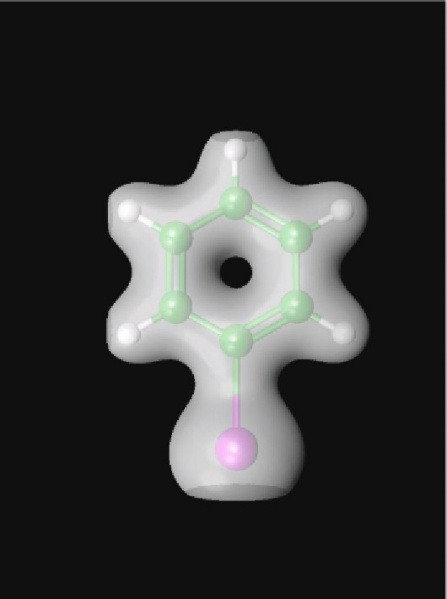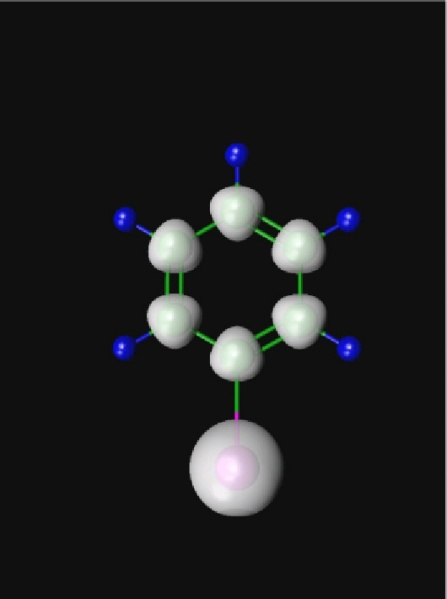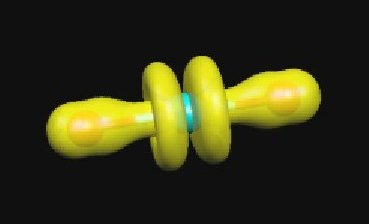*DENSITY¶

The density can be dumped to formatted file in CUBE file format for subsequent visualization (e.g. using Molekel or MOLDEN or other programs; see also POV-Ray).

The large and small component density is written to files rhol.cube and rhoS.cube, respectively. They can be recovered from the work area by the command

pam -get rhoL.cube rhoS.cube ...

Note also that the coefficient file DFCOEF must be present in order to generate the density.

pam --incmo ...

Large component density of iodobenzene plotted using Molekel (cutoff: 0.01):Small component density of iodobenzene plotted using Molekel (cutoff: 0.0001):It is also possible to plot the density of an orbital by subtracting densities generated from a given coefficient file, varying the occupation. The figure shows the large component density of the HOMO of the uranyl cation obtained by subtracting from the density generated from regular occupation the density generated by reducing the occupation by two electrons..ORBITALS¶

This keyword is only available in the Development version of Dirac. Make individual cube files for each give orbital, instead of the total density.

Example:

.ORBITALS
4..9
1,3

This generates cube files for gerade orbitals 4-9 and ungerade orbitals 1 and 3. The numbering starts from the first occupied orbital, so “positronic” orbitals have negative indices. Be aware that the cube generation can be rather slow for large basis sets. Writing hundreds of cube files can take much more time than a SCF calculation.

.DOCUBE¶

Specify large/small component density generation (1 = on; 0 = off).

Default:

.DOCUBE
1 1

.NCUBE¶

Number of cells along the sides of the cube.

Default:

.CUBE
80 80 80

Adjust the volume of the cube.

Default:

4.0 8.0

DIRAC will look at atomic positions and then try to place the cube such that most density is included. The origin of the cube is determined by finding the minimum atomic position in the x-, y-and z-direction separately and then add CUBADJ(1). The length of the vectors spanning the cube (parallelepiped) are analogously defined by maximal atomic positions, with CUBADJ(2) added.

Print level.

Default:

.PRINT
0